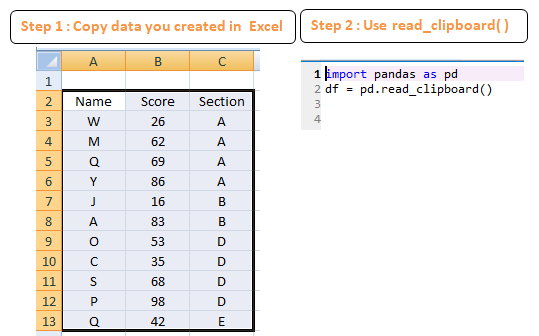# Create Dummy Data in Python

This article explains various ways to create dummy or random data in Python for practice. Like R, we can create dummy data frames using pandas and numpy packages. Most of the analysts prepare data in MS Excel. Later they import it into Python to hone their data wrangling skills in Python. This is not an efficient approach. The efficient approach is to prepare random data in Python and use it later for data manipulation.

## 1. Enter Data Manually in Editor Window

The first step is to load pandas package and use `DataFrame `function
```import pandas as pd
data = pd.DataFrame({"A" : ["John","Deep","Julia","Kate","Sandy"],
"MonthSales" : [25,30,35,40,45]})```
```       A  MonthSales
0   John          25
1   Deep          30
2  Julia          35
3   Kate          40
4  Sandy          45
```

Note : Character values should be defined in single or double quotes.

If you notice the syntax of pandas dataframe, columns and row values are defined in dictionary. If you understand the concept of dictionary, you wouldn't need to mug up where to add `{ }` and `[ ]`.
Syntax of Dataframe : pd.DataFrame(dictionary)
```d = {"A" : ["John","Deep","Julia","Kate","Sandy"],
"MonthSales" : [25,30,35,40,45]}
pd.DataFrame(d)
```

## 2. Read Data from Clipboard

In general, MS Excel is the favorite reporting tool of analysts especially when it comes to creating dummy data. People generally prefer entering data in Excel and pasting it to Python for creating data frame. In pandas, there is an option to import data from clipboard (i.e. copied data) using read_clipboard( ) function from pandas package.Read copied data in Python

```import pandas as pd
```
```   Name  Score Section
0     W     26       A
1     M     62       A
2     Q     69       A
3     Y     86       A
4     J     16       B
5     A     83       B
6     O     53       D
7     C     35       D
8     S     68       D
9     P     98       D
10    Q     42       E
```

## 3. Entering Data into Python like SAS

Similar to SAS, we can input data in editor window with delimiter or separator. We can use any separator - comma, space, tab etc.
```import pandas as pd
from pandas.compat import StringIO

text = """
Name,Score,Section
W,26,A
M,62,A
Q,69,A
"""
```
```import pandas as pd
from pandas.compat import StringIO

text = """
Name Score Section
W 26 A
M 62 A
Q 69 A
"""

```
`\s+` means one or more space as a separator at time of reading data.

## 4. Prepare Data using sequence of numeric and character values

Let's import two popular python packages for this task - string and numpy. The package string is used to generate series of alphabets. Whereas numpy package is used to generate sequence of numbers incremented by a specific value.
```import pandas as pd
import string
import numpy as np
data2 = pd.DataFrame({"A": np.arange(1,10,2),
"B" : list(string.ascii_lowercase)[0:5],
})
```
```   A  B
0  1  a
1  3  b
2  5  c
3  7  d
4  9  e
```

Explanation
1. `np.arange(1,10,2)` tells python to generate values between 1 and 10, incremented by 2.
2.`string.ascii_lowercase` returns abcdefghijklmnopqrstuvwxyz. list(string.ascii_lowercase)[0:5] is used to pick first 5 letters.

## 5. Generate Random Data

In numpy, there are many functions to generate random values. The two most popular random functions are `random.randint( )` and `random.normal( )`
```import pandas as pd
import numpy as np
np.random.seed(1)
data3 = pd.DataFrame({"C" : np.random.randint(low=1, high=100, size=10),
"D"  : np.random.normal(0.0, 1.0, size=10)
})
```
```    C         D
0  38 -0.528172
1  13 -1.072969
2  73  0.865408
3  10 -2.301539
4  76  1.744812
5   6 -0.761207
6  80  0.319039
7  65 -0.249370
8  17  1.462108
9   2 -2.060141
```

Explanation
`np.random.seed(1)` tells python to generate same random values with this seed when you run it next time. `np.random.randint(low=1, high=100, size=10)` returns 10 random values between 1 and 100. `np.random.normal(0.0, 1.0, size=10)` returns 10 random values following standard normal distribution having mean 0 and standard deviation 1.

Check mean and standard deviation of normal distribution
np.round(np.std(np.random.normal(0.0, 1.0, size=1000)))
np.round(np.mean(np.random.normal(0.0,1.0, size=1000)))

Generate more than 1 random variable with a single function
`np.random.randn(6, 4)` tells Python to generate 6 random values from the "standard normal distribution" in 4 columns
```np.random.seed(12)
df = pd.DataFrame(np.random.randn(6, 4),
index=list('abcdef'), columns=list('ABCD'))
```
```          A         B         C         D
a  0.472986 -0.681426  0.242439 -1.700736
b  0.753143 -1.534721  0.005127 -0.120228
c -0.806982  2.871819 -0.597823  0.472457
d  1.095956 -1.215169  1.342356 -0.122150
e  1.012515 -0.913869 -1.029530  1.209796
f  0.501872  0.138846  0.640761  0.527333
```

## 6. Create Categorical Variables

In this step, we will create two types of categorical variables :
• Categories ranging from 1 to 4
• Binary variable (0 / 1)
```import pandas as pd
import numpy as np
np.random.seed(1)
data4 =pd.DataFrame({"X"  : np.random.choice(range(1,5), 20, replace=True),
"X1" : np.where(np.random.normal(0.0, 1.0, size=20)<=0,0,1)})
```
```    X  X1
0   2   1
1   4   0
2   1   1
3   1   0
4   4   1
5   2   0
6   4   0
7   2   0
8   4   1
9   1   0
10  1   0
11  2   0
12  1   1
13  4   1
14  2   0
15  1   1
16  3   1
17  2   1
18  3   1
19  1   0
```

Explanation
1. np.random.choice(range(1,5), 20, replace=True) means generating 20 values from 1 to 4 (excluding 5) with replacement (i.e. repeated values).
2. np.where(np.random.normal(size=20)<=0,0,1) implies if random value is either zero or negative, make it 0. Otherwise 1. np.where( ) is used to construct IF-ELSE statement in python.
Like R's factor( ) function, you can define variable(s) as categorical variables. See the code below.
data4.X  = data4.X.astype("category")
data4.X1 = data4.X1.astype("category")

## 7. Import CSV or Excel File

Using pandas functions read_csv( ) and read_excel( ) functions, you can read data from excel or CSV to Python.
import pandas as pdShare
Related Posts1.